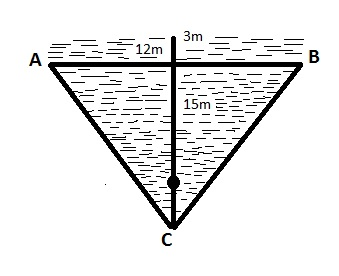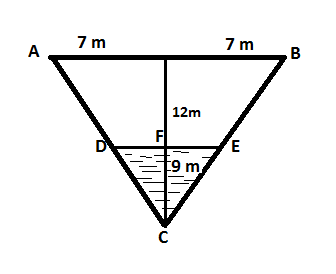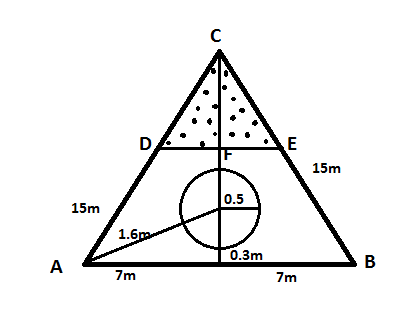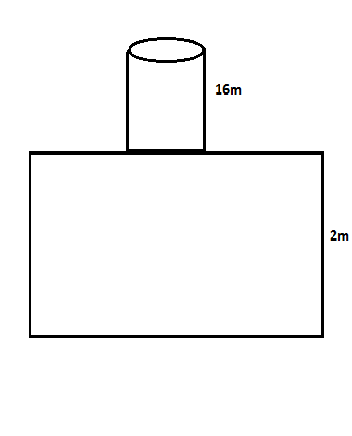Staility – I : Chapter 2 |

# Staility – I : Chapter 2

1. ###### Find the thrust exprienced by a flat keel plate 10m x 2m when the draft is 8m in SW.

Solution :  Area of the flat keel plate = (10 x 2)
= 20m2
Pressure = (depth x density)
=    (8 x 1.025)
=  8.2t /m2
Thrust = (pressure x area)
= 8.2 x 20 =164 t .

1. ###### A box-shaped vessel 150m x20m x 12m is floating in a dock of RD 1.010 at an even keel draft of 10m. Find the total water pressure experienced by the hull.

Solution: The total water pressure exerted on the hull to the water =  (Thrust of keel plate which acting   horizontally + Thrust of forward and aft  which act vertically +Thrust of  port and stbd side which act vertically)
Thrust of Keel plate = (pressure x area)
Pressure = (depth x density)
=10 x1.010 t/m2

Area = 150m x 20m = 3000m2
Thrust =(10 x1.010 x 3000) = 30, 300 t

Thrust of forward and Aft =(pressure x area)

Pressure = depth x density
=( 5 x1.010)t/m2

Area =L X B
=(20 x10)
= 200m2

Thrust = (P x A)
= (5×1.010 x200)
= 1010t

Thrust acting both forward and aft = (1010 x2)
= 2020 t

Thrust of port and stbd  side = (pressure x area)

Pressure  =( depth x density)
=( 5×1.010)

Area = (L X B)
= 150m x 10 = 1500m2

Thrust = ( P X A )
= 5 x 1.010 x1500
= 7575 t

Thrust acting on Both sides = 7575 x 2
=15150 t

Hence , Total pressure on the hull = (30300 +2020 + 15150)
=47,470 tonnes.

1. A submarine has a surface area of 650m2 and can with stand a total water pressure of 1332500 t . Find at what approximate depth in SW she would collapse.

Solution:     Area = 650m2
Thrust = 1332500t
RD = 1.025
Depth =?

Thrust = ( P x A )
1332500 = (P x 650)
P = 2050 t/m2

Now,  P =(depth x density)
2050 = (depth x 1.025)

Hence ,  Depth = 2000m.

1. ###### A rectangular lock gate 40m wide and 20m high has water of RD 1.010 12m deep on one sideand water of RD 1.020 11m deep on the other. Find the resultant thrust experienced and direction in which it acts.

Solution: Water of RD =1.010
Depth =12 m
C = (12/2)
=6m

Pressure = (depth x density)
=(6 x1.010) t/m2

Thrust =(P x A)  =( 6 x 1.010 x40 x12)
= 2908.8t

Water of RD = 1.020
Depth = 11m
C = (11/2)
=5.5m

Pressure =(depth x density)
= (5.5 x 1.020 ) t/m2

Area =( L X B) =(40 x 11)
= 440m2

Thrust =( P X A) = (5.5 x 1.020 x 440)
= 2468.4 t

Thus we can Resultant thrust =(2908.8 – 2468.4)
= 440.4t

1. ###### A rectangular lock gate 36m wide and 20m high has FW on one side to a depth of 16m. Find what depth of SW on the other side will equalize the thrust.

Solution Inside the lock gate area =( 36 x 16)m2

Pressure = depth x density =(16/2 x 1)
= 8 t/m2

Thrust inside the lock gate = (pressure x area)
Thrust =(8 x 36 x 16)
= 4608 t

Let ‘X’ depth of   salt water on other side will equalize the thrust .

Pressure     = depth x density =( X/2 x 1.025)
Area    =(36 x  X )
Thrust = (P x A)

4608 = (X/2 x 1.025 x 36X )
4608 = (1.025 x 36X  x  X/2)
4608 =   (1.025 x 36X2/2)
=   ( 1.025 x 18X2)
X2   = (4608 /1.025 x 18)

X = 15.8 m

Hence, Depth of other side of lockgate =15.8m

###### Solution :

Height       = 15m
Pressure   = (depth x density)
Thrust       = (pressure x area)
Height of the water inside the tank = 15mWater pressed up to ahead of  = 3m
‘C’ inside the tank = (1/3 x15)
= 5 m
Outside the tank =    3m
so total =5 + 3 =8m

Now pressure =(depth x density)

=( 8 x 1.025)

Area = (1/2 x 12 x15)
= 90m2

Thrust  experienced  = (pressure x area)
= (90 x 8 x 1.025)
=738t.

###### Solution :

B = 14m, H =12mAs we know that :
Pressure = (depth x density)
Thrust   = (pressure x area)

An right angle triangle PBC , PB =7m
Since, Height divide AB  equally into two parts.
Triangle PBC  and   FEC are similar
So by law of similar angle triangle

PB/ FE = PC / FC
7 / FE  =12 / 9

Hence, FE  =  (63 / 12)
= 5.25m

In triangle DEC
DF = FE
DE = (2 X FE)
= 2X 5.25
=10.5m

Area of triangle DEC = (1/2 x10.5×9)
=47.25m2

Pressure = (depth x density)
= (9 x 1/3 x 1.025)
= 3.075 t/m2

Hence Thrust experienced  = (P X A)
= (3.075 x 47.25)
=145. 29 t

###### Solution:

Base = 14m , Sides = 15m , Depth of the oil = 10mPressure = (depth x density)
=  (10 – 0.8 x 0.95)
= 8.74 t/m2

Area of the circle = π r2
= (3.1416 x 0.5 x 0.5)
=0.7854m2

Thrust = (P X A)
=( 8.74 x 0.7854)
= 6.864 t.

###### Solution :

Rectangular deep tank  = 22m x 20m x10m
Trunk way = (5m x 4m x 0.2m)Pressure = (depth x density)
= (2.64m – 0.2) x1.025

Since , Depth is (2.64m – 0.2 )  and we have the calculate the thrust   act  on the trunkway and the water level above the trunkway will act as thrust )

If this trunkway would have been placed inside the tank then the depth will be   (10m + 2.64m – 0.2/2m ).

Area of the trunkway = (L X B)
= 4×5
=20 m2

Thrust experienced = (P X  A)
= (2.501 x 20 )
= 50.02tonnes.

###### Solution :

Volume of  tank  = (L X B X H)
= (25m x 20m x 2m)

Area    =     (25 x20)
=   500m2Pressure = (depth x density)
= (16 x 1.025)
=16.4  t/m2

Thrust on tank top  = (PX A)
= (16.4 X 500)
= 8200 t

Pressure on the tank bottom = (depth x density)
= (18 x1.025)              = 8.45t/m2

Thrust   act  on the tank bottom = (P X A)
=   (500 x 18 .45)
= 9225t

Pressure act on outside of the tank bottom = (depth x density)
= (10 x 1.025)
= 10.25t

Hence, Thrust acting = (P X A)
= (10.25 X500)
= 5125 tonnes.

Finally, Resultant thrust = (9225 -5125)
= 4100 tonnes.•rasel says: